Publicité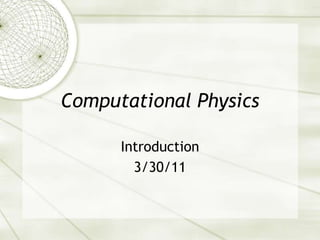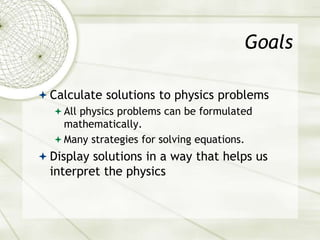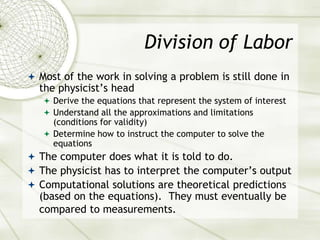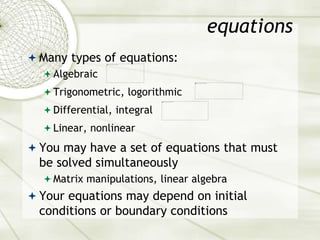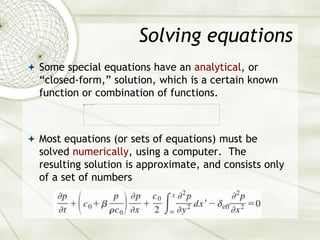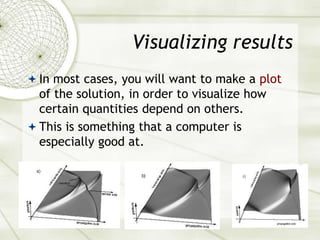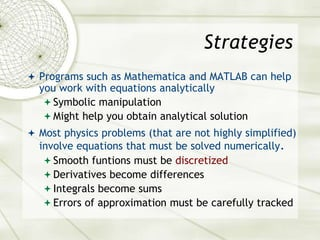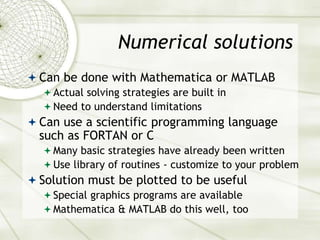1 sur 8
Publicité

### intro.ppt

1. Computational Physics Introduction 3/30/11
2. Goals  Calculate solutions to physics problems  All physics problems can be formulated mathematically.  Many strategies for solving equations.  Display solutions in a way that helps us interpret the physics
3. Division of Labor  Most of the work in solving a problem is still done in the physicist’s head  Derive the equations that represent the system of interest  Understand all the approximations and limitations (conditions for validity)  Determine how to instruct the computer to solve the equations  The computer does what it is told to do.  The physicist has to interpret the computer’s output  Computational solutions are theoretical predictions (based on the equations). They must eventually be compared to measurements.
4. equations  Many types of equations:  Algebraic  Trigonometric, logorithmic  Differential, integral  Linear, nonlinear  You may have a set of equations that must be solved simultaneously  Matrix manipulations, linear algebra  Your equations may depend on initial conditions or boundary conditions
5. Solving equations  Some special equations have an analytical, or “closed-form,” solution, which is a certain known function or combination of functions.  Most equations (or sets of equations) must be solved numerically, using a computer. The resulting solution is approximate, and consists only of a set of numbers
6. Visualizing results  In most cases, you will want to make a plot of the solution, in order to visualize how certain quantities depend on others.  This is something that a computer is especially good at.
7. Strategies  Programs such as Mathematica and MATLAB can help you work with equations analytically  Symbolic manipulation  Might help you obtain analytical solution  Most physics problems (that are not highly simplified) involve equations that must be solved numerically.  Smooth funtions must be discretized  Derivatives become differences  Integrals become sums  Errors of approximation must be carefully tracked
8. Numerical solutions  Can be done with Mathematica or MATLAB  Actual solving strategies are built in  Need to understand limitations  Can use a scientific programming language such as FORTAN or C  Many basic strategies have already been written  Use library of routines - customize to your problem  Solution must be plotted to be useful  Special graphics programs are available  Mathematica & MATLAB do this well, too
Publicité